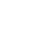# Draw more complex 3D Models using Triangle Fans in WebGL

Published 5 years ago
Updated 3 years ago

This time we look at the last drawing mode, triangle fan, which can be useful for drawing different types of 3d forms. Combine this mode with triangle strip to creating complex models.

[00:00] Let's take a look at the last drawing mode available for the draw/erase function, GL_TRIANGLE_FAN. The name itself should give you a rough idea of what it does. If you add any paper folded fan, not an electrical rotary fan.

[00:14] Say we have groups of points like this. The first point, point zero at the top, and the rest spread left to right across the bottom. A triangle fan takes points zero, one, and two, and creates the first triangle, just like both of the other triangle drawing methods. Then, it goes to zero, two, three, then zero, three, four, and so on.

[00:35] The fan always has that initial starting point that connects all the triangles. It uses that initial point, plus each successive pair of points, to create each successive triangle. In a fan, every triangle shares an edge with at least one of the triangle, and all triangles share that initial point.

[00:52] Let's see it in action. I'll start with the code we had for the triangle strip example. First, I'll create a center point, we can put all seven values into the array with one push. It will be at zero, zero, zero, and the color will be zero, zero, zero with one for alpha, solid black.

[01:09] Now, I'll make this for loop go from zero to math pi*2 radians which is equivalent to 0to 360 degrees. I'll increment by 01, and I'll arrange the vertices around in a circle, using the same easy trick. X will be math cosine I, and Y will be math sine I. Z will be zero for now, and I'll set the colors with random values for red, green, and blue, plus one for alpha.

[01:37] Down to the draw function, I'll just change this to points, just to see what we have. Yep, we have the center black point, and the rest arranged in a circle around that center point.

[01:49] Let's change this to fan mode. You see we have a solid circle shape, you might notice that it's not quite closed though, we can handle that later. We can now do some more modeling with these vertices. Instead of being flat, let's change the Z positions somehow.

[02:07] Let's have them go up and down in a sine wave. I'll say Z to math sine i1001 OK. That's more interesting. Now, the colors, for red, I'll use the same sine wave, but I'll multiply it by 05, so, it goes from -05 to +05. Then I'll add 05 to that, so, it arranges from 0to 1, which is a valid range for the color channels here. I'll do the same thing for green and blue, but I'll have them use i8, and i12 just to get a variation. Cool, these colors now flow nicely together.

[02:49] Let's handle that little gap. I'll copy all the points here, and paste them down again, after the for loop. I'll save before that, i=math pi*2, that will pull one final point where it needs to be a closer circle. Looks good.

[03:07] Now, to me this looks like some kind of shell, let's roll with that theme. I am going to try to move the center point up a bit, 09 on the Y-axis, and 03 on the Z-axis. I'll change the center color to white. Now, it looks even more like a shell, let's keep going.

[03:26] I'll up the resolution by changing the increment to the for loop, then I'll set the clear color to a dark blue. Now, we've got something pretty cool going on. With triangle strip and fans you can start creating some pretty complex models. See what you can do with them.Bojan
~ 5 years ago

Hi Keith,

I love your tutorials and have been following them very closely. In your tutorial you copied the last point underneath the for loop. Wouldn't it be better to write i <= Math.PI * 2 in the loop itself?Keith Petersinstructor
~ 5 years ago

The thing is, you are adding a small amount to a variable over time. It's unlikely that it's every going to exactly equal Math.PI * 2 which is 6.283185307179586. In fact, I can almost guarantee that your incremented variable will never come out to exactly that value. If you were using whole numbers like a loop from 0 to >= 10 and incrementing by 1, then yeah, you'd reach 10. It's different with floating point numbers.